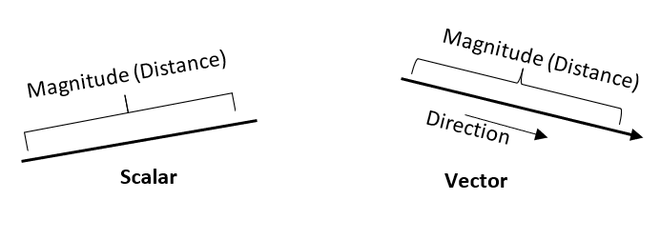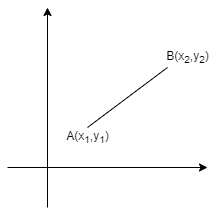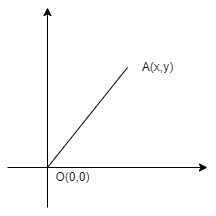Open in App
Not now

# What is the Magnitude of a Vector Formula?

• Last Updated : 01 Feb, 2022

A vector has both direction and magnitude. The magnitude of a vector is the length of the vector. It summarizes the numeric value of the vector. The magnitude of any vector is always positive. Velocity, displacement, momentum, force, etc. come under vectors. It summarizes the individual measures of the vector along the x-axis, y-axis, and z-axis if it is three-dimensional.The above figure is a pictorial representation that depicts the differences between scalar quantity and vector quantity.

### Magnitude of a Vector Formula

There are different ways to calculate the magnitude of the vector. Based on the given data, use a different kind of formula to find the magnitude of a vector. Following are the ways to calculate the magnitude. The magnitude of a vector A is represented using the modulus operator i.e., |A|

• If they are given a vector Ā = xi+ yĵ + zk then the magnitude of vector Ā can be calculated using the below formula

Magnitude of vector Ā (|A|) =• If the starting point and endpoint of a vector is given i.e., (x1, y1) and (x2, y2) respectively like specified in the below pictureVector with start and end points

The magnitude of a vector when the start and endpoints of a vector given are nothing but the distance between the points. The formula for finding magnitude if given by-

|Ā|=• If any of the starting or endpoint of a vector is at origin o(0, 0) and another point A(x, y) like specified in the below figure,vector with one end at the origin

Then the formula for finding the magnitude of vector where one of end of a vector is at origin is given by-

|Ā| = √(x2+y2)

Where x, y are data points

Let’s look into a few examples to get a clear view of this.

### Sample Problems

Question 1: What is the magnitude for the vector Ā = 2i + 3ĵ + 4k?

Solution:

Given vector Ā = 2i + 3ĵ + 4k

Magnitude |A| == √29

= 5.38

The magnitude of vector 2i+ 3ĵ + 4k is 5.38

Question 2: What is the magnitude for the vector Ā = 3i + 3ĵ – 6k?

Solution:

Given vector Ā = 3i + 3ĵ – 6k

Magnitude |A| == √54

= 7.35

The magnitude of vector 3i+ 3ĵ – 6k is 7.35.

Question 3: Find the magnitude of the vector if the starting point of a vector is (3, 4) and the ending point is (6, 2).

Solution:

Given,

(x1, y1) = (3, 4)

(x2, y2) = (6, 2)

|Ā|== √(32+(-2)2)

= √(9+4)

= √13

= 3.6

So the magnitude of the vector with given starting and end points is 3.6

Question 4: What is the magnitude of the vector if the starting point of a vector is (2, 1, 4) and the ending point is (5, 2, 6).

Solution:

Given,

(x1, y1, z1) = (2, 1, 4)

(x2, y2, z2) = (5, 2, 6)

|Ā| == √(9 +1 + 4)

= √14

= 3.74

So the magnitude of the vector with given starting and end points is 3.74

Question 5: What is the magnitude of the vector which starts at the origin and endpoint at (3, 4).

Solution:

Given

Starting point of vector is at origin O(0, 0)

End point (x, y) = (3, 4)

From the above formula Magnitude of vector (|Ā|) = √(x2+y2)

= √(32 + 42)

= √(9 + 16)

= √25 = 5

So magnitude for the vector with one of end point at origin is 5

Question 6: Find the magnitude of the vector which one of the endpoints is at origin and the other point at (1, 4, 3).

Solution:

Given,

One of the end point of vector is at origin O(0, 0)

Other point (x, y) = (1, 4, 3)

From the above formula Magnitude of vector (|Ā|) = √(x2+y2+z2)= √26 = 5.09

So magnitude for the vector with one of end point at origin is 5.09

My Personal Notes arrow_drop_up
Related Articles